# Non Verbal Reasoning - Classification

### Exercise :: Classification - Section 1

In each problem, out of the five figures marked (1), (2), (3), (4) and (5), four are similar in a certain manner. However, one figure is not like the other four. Choose the figure which is different from the rest.

6.

Choose the figure which is different from the rest.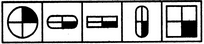(1)     (2)     (3)     (4)     (5)

 A. 1 B. 2 C. 3 D. 4 E. 5

Explanation:

In all other figures, the lower-right quarter portion is shaded.

7.

Choose the figure which is different from the rest.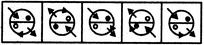(1)     (2)     (3)     (4)     (5)

 A. 1 B. 2 C. 3 D. 4 E. 5

Explanation:

Only in fig. (5), the arrowhead along the circumference of the circle indicates motion in an ACW direction.

8.

Choose the figure which is different from the rest.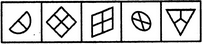(1)     (2)     (3)     (4)     (5)

 A. 1 B. 2 C. 3 D. 4 E. 5

Explanation:

All other figures are divided into equal parts.

9.

Choose the figure which is different from the rest.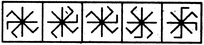(1)     (2)     (3)     (4)     (5)

 A. 1 B. 2 C. 3 D. 4 E. 5

Explanation:

Figure (1) and fig. (3) can be rotated into each other and fig. (4) and fig. (5) can be rotated into each other.

10.

Choose the figure which is different from the rest.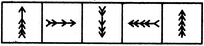(1)     (2)     (3)     (4)     (5)

 A. 1 B. 2 C. 3 D. 4 E. 5